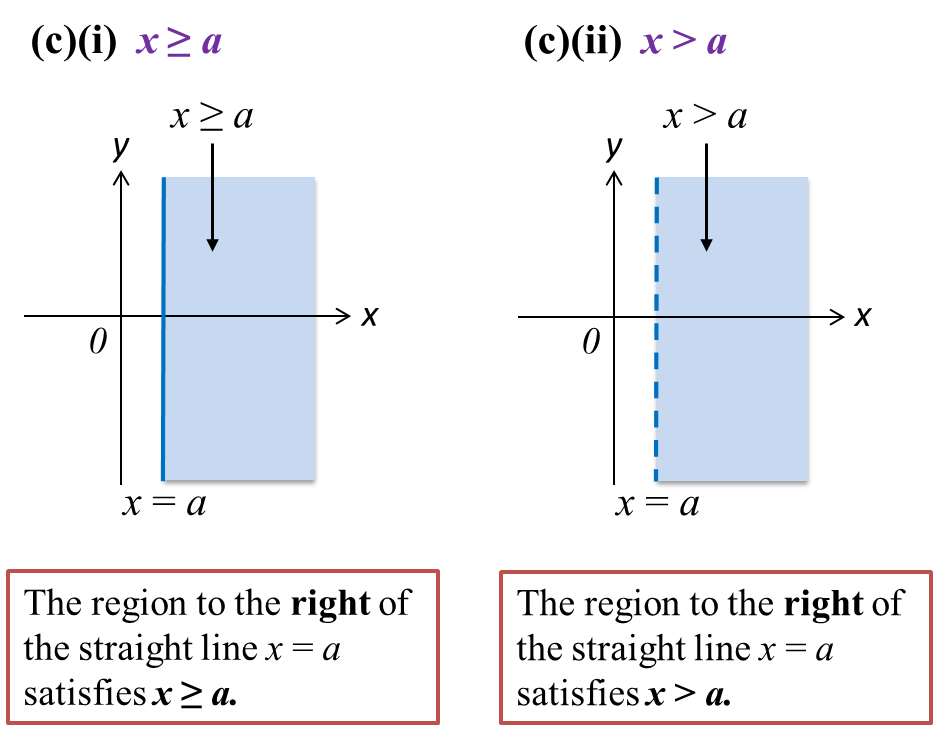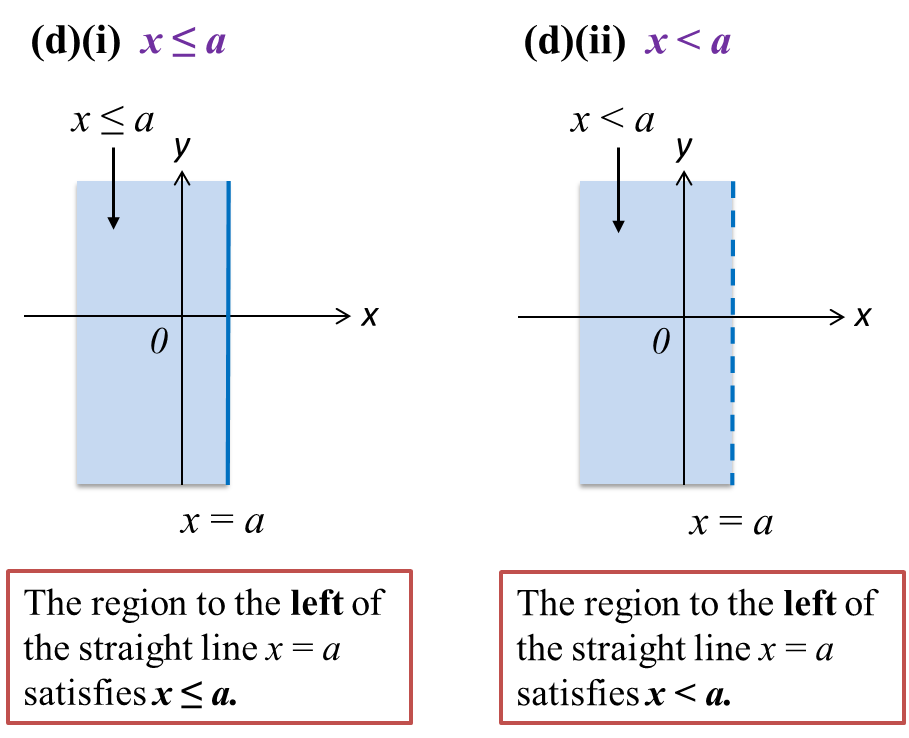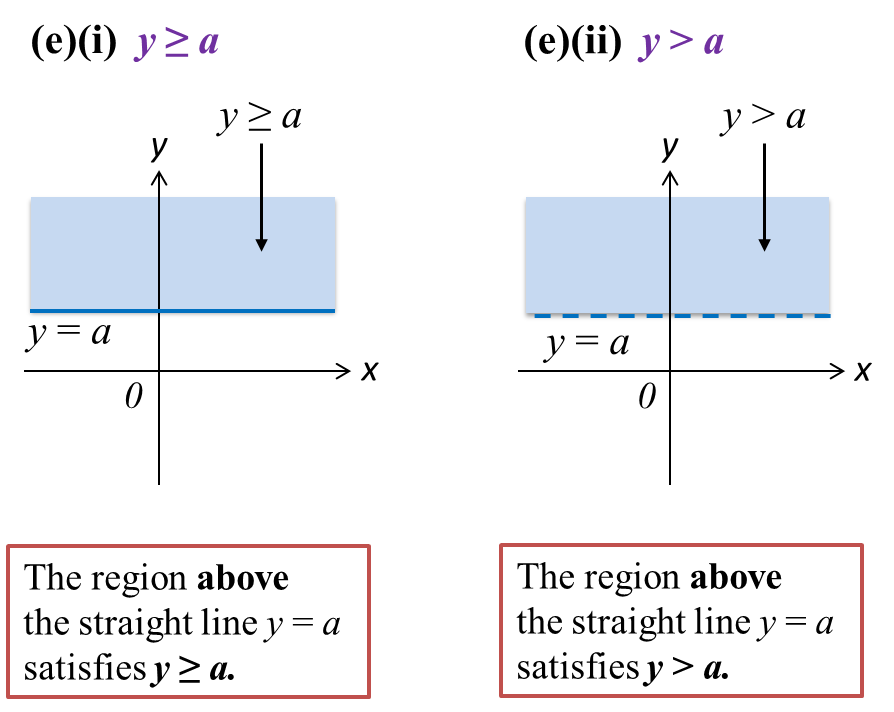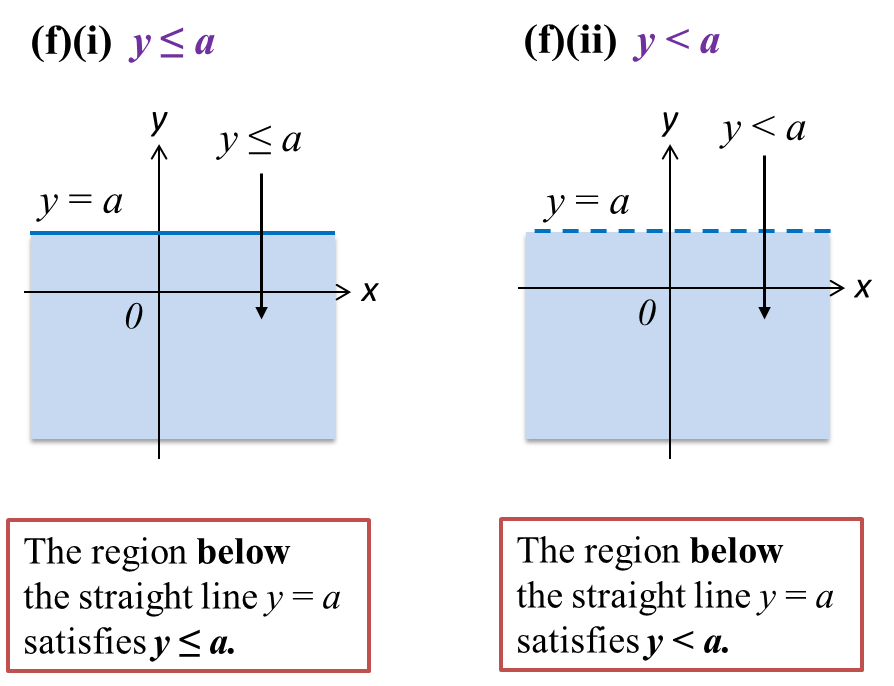# 6.1 Linear Inequalities in Two Variables

6.1.1 Position of a point relative to the graph of y = ax+ b
1. When = ax + b, the point is on the line.
2. When < ax + b, the point is below the line.
3. When > ax + b, the point is above the line.

6.1.2 Identifying the region satisfying the respective inequalities.

1.   A dashed line ‘----’ is used when points on the line are not included in the region representing the inequality, such as y > ax + b or y < a or x > a.

2.   A solid line ‘___’ is used when points on the line are not included in the region representing the inequality, such as yax + b or y ≤  a or xa.

3.   The diagrams below show the regions that satisfy the respective inequalities.